#The Complex Plane#### Complex numbers are points in the plane

In the same way that we think of real numbers as being points on a line, it is natural to identify a complex number z=a+ib with the point (a,b) in the cartesian plane. Expressions such as ``the complex number z'', and ``the point z'' are now interchangeable.

We consider the a real number x to be the complex number x+ 0i and in this way we can think of the real numbers as a subset of the complex numbers. The reals are just the x-axis in the complex plane.

The modulus of the complex number z= a + ib now can be interpreted as the distance from z to the origin in the complex plane.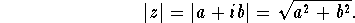Since the hypotenuse of a right triangle is longer than the other sides, we have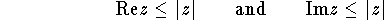for every complex number z.

We can also think of the point z= a+ ib as the vector (a,b). From this point of view, the addition of complex numbers is equivalent to vector addition in two dimensions and we can visualize it as laying arrows tail to end. (Picture)

We see in this way that the distance between two points z and w in the complex plane is |z-w|.

Exercise: Prove this last statement algebraically. (Proof.)

Exercise: Prove the ``Parallellogram law''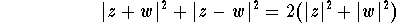(Proof.)

The ``Triangle'' inequality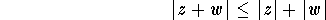is easily seen to hold.
(Proof.)

Exercise: Prove the Triangle inequality for n complex numbers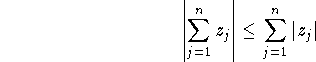Here are some more exercises.[Algebra] [Complex Variables]
[Geometry] [Trigonometry ]
[Calculus] [Differential Equations] [Matrix Algebra]S.O.S MATHematics home page

Do you need more help? Please post your question on our S.O.S. Mathematics CyberBoard.Author: Michael O'Neill